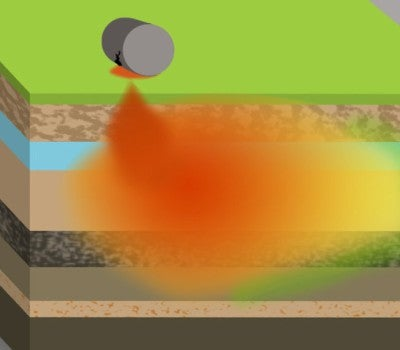# Online Courses for College Students and Lifelong LearnersWe will build upon basic environmental sciences and environmental engineering principles to discover how to best implement MNA as a viable treatment for groundwater contamination plumes. Additionally ...Learn core topics in oral communication....Study of ordinary differential equations (e.g., solutions to separable and linear first-order equations and to higher-order linear equations with constant coefficients, systems of linear differential ...Study of ordinary differential equations (e.g., solutions to separable and linear first-order equations and to higher-order linear equations with constant coefficients, systems of linear differential ...This course teaches industry professionals and students the fundamental concepts of parallel programming in the context of Java 8. Parallel programming enables developers to use multicore computers to...Parallel, concurrent, and distributed programming underlies software in multiple domains, ranging from biomedical research to financial services. This specialization is intended for anyone with a basi...This is course 3 in the Leadership Development for Engineers Specialization and part of Rice University’s Engineering Management and Leadership Certificate. An actionable leadership improvement plan e...Learn the physics of how things move with this calculus-based course in Mechanics! PHYS101x serves as an introduction to mechanics following a standard first semester university physics course. This...The course begins with kinematics, where you will learn to use equations and graphs to describe the position, velocity, and acceleration of an object, and how those quantities are related through calc...This course serves as an introduction to the physics of energy and momentum. Upon completion, learners will have an understanding of how mathematical laws and conservation principles describe the mot...This course serves as an introduction to the physics of force and motion. Upon completion, learners will have an understanding of how mathematical laws and conservation principles describe the motion...This course serves as an introduction to the physics of rotational motion and gravitation. Upon completion, learners will have an understanding of how mathematical laws and conservation principles de...

Body